Скачать презентацию Chapter 10 Interest Rates Chapter outline

cbf7758a9231ca057d5670d4fadc4186.ppt

• Количество слайдов: 26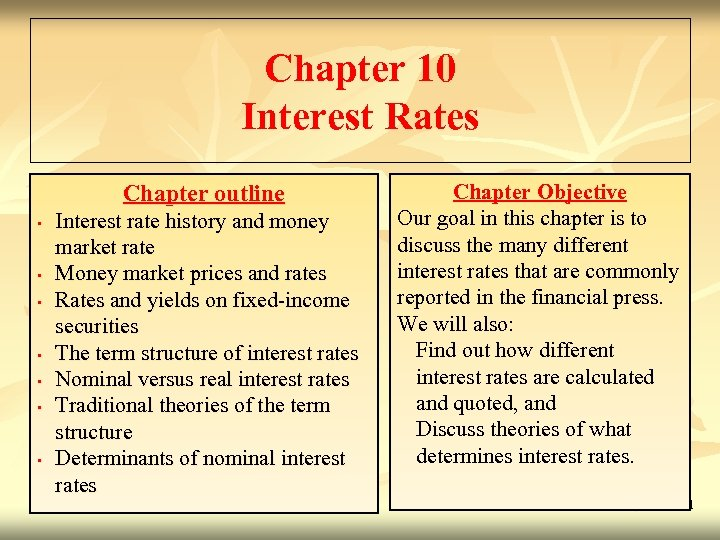Chapter 10 Interest Rates Chapter outline • • Interest rate history and money market rate Money market prices and rates Rates and yields on fixed-income securities The term structure of interest rates Nominal versus real interest rates Traditional theories of the term structure Determinants of nominal interest rates Chapter Objective Our goal in this chapter is to discuss the many different interest rates that are commonly reported in the financial press. We will also: Find out how different interest rates are calculated and quoted, and Discuss theories of what determines interest rates. 11 - 1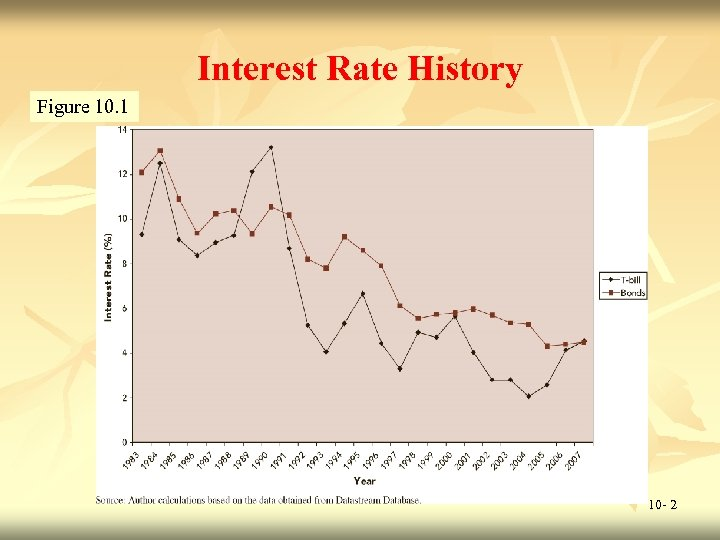Interest Rate History Figure 10. 1 10 - 2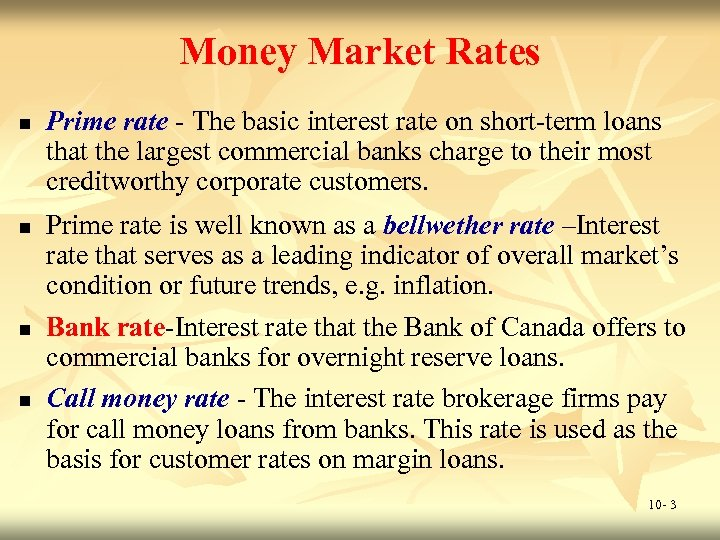Money Market Rates n n Prime rate - The basic interest rate on short-term loans that the largest commercial banks charge to their most creditworthy corporate customers. Prime rate is well known as a bellwether rate –Interest rate that serves as a leading indicator of overall market’s condition or future trends, e. g. inflation. Bank rate-Interest rate that the Bank of Canada offers to commercial banks for overnight reserve loans. Call money rate - The interest rate brokerage firms pay for call money loans from banks. This rate is used as the basis for customer rates on margin loans. 10 - 3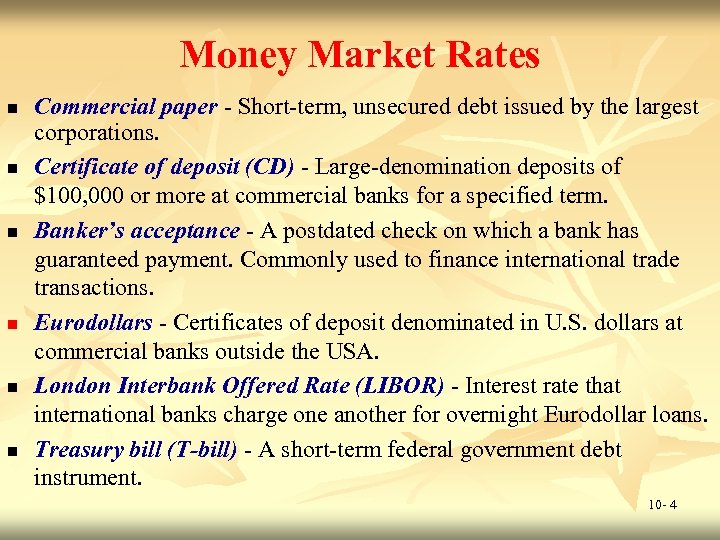Money Market Rates n n n Commercial paper - Short-term, unsecured debt issued by the largest corporations. Certificate of deposit (CD) - Large-denomination deposits of \$100, 000 or more at commercial banks for a specified term. Banker’s acceptance - A postdated check on which a bank has guaranteed payment. Commonly used to finance international trade transactions. Eurodollars - Certificates of deposit denominated in U. S. dollars at commercial banks outside the USA. London Interbank Offered Rate (LIBOR) - Interest rate that international banks charge one another for overnight Eurodollar loans. Treasury bill (T-bill) - A short-term federal government debt instrument. 10 - 4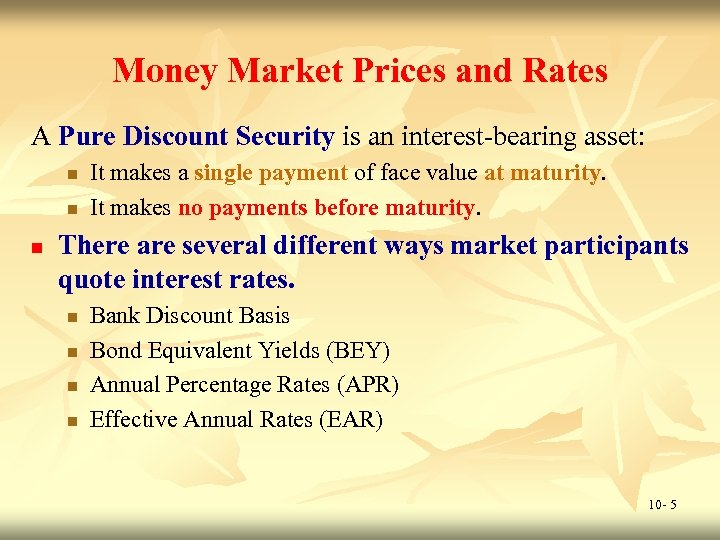Money Market Prices and Rates A Pure Discount Security is an interest-bearing asset: n n n It makes a single payment of face value at maturity. It makes no payments before maturity. There are several different ways market participants quote interest rates. n n Bank Discount Basis Bond Equivalent Yields (BEY) Annual Percentage Rates (APR) Effective Annual Rates (EAR) 10 - 5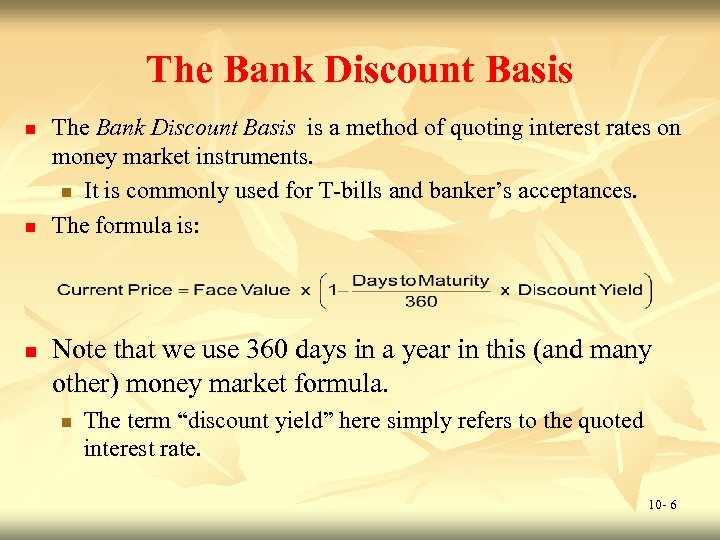The Bank Discount Basis n n n The Bank Discount Basis is a method of quoting interest rates on money market instruments. n It is commonly used for T-bills and banker’s acceptances. The formula is: Note that we use 360 days in a year in this (and many other) money market formula. n The term “discount yield” here simply refers to the quoted interest rate. 10 - 6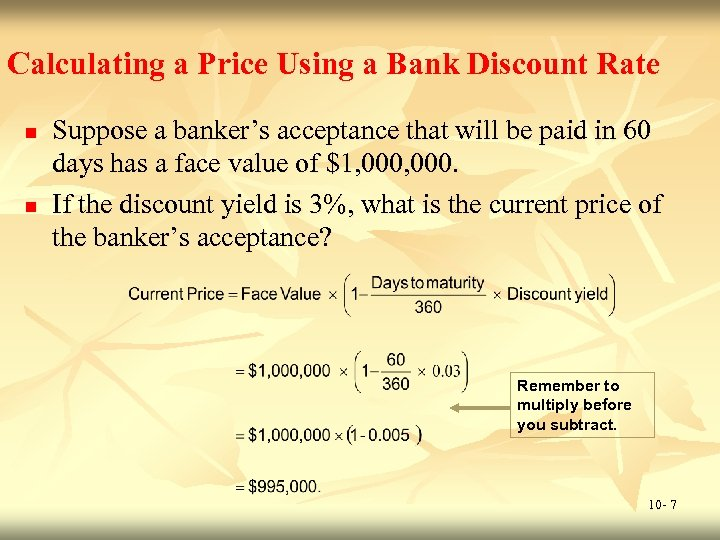Calculating a Price Using a Bank Discount Rate n n Suppose a banker’s acceptance that will be paid in 60 days has a face value of \$1, 000. If the discount yield is 3%, what is the current price of the banker’s acceptance? Remember to multiply before you subtract. 10 - 7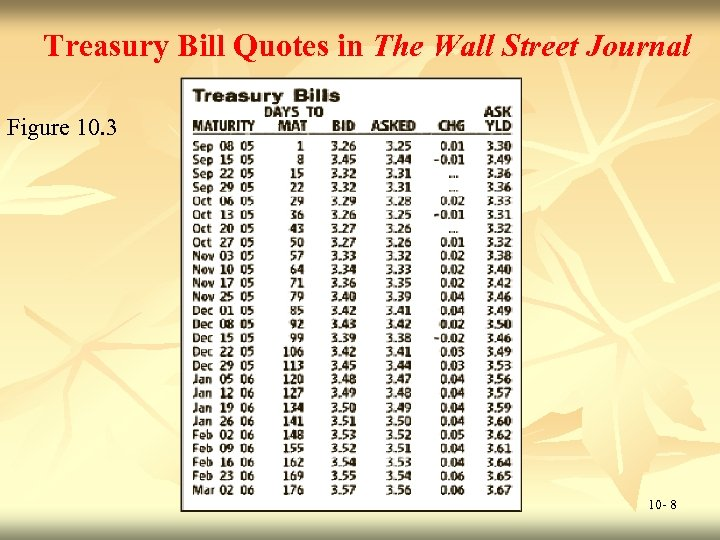Treasury Bill Quotes in The Wall Street Journal Figure 10. 3 10 - 8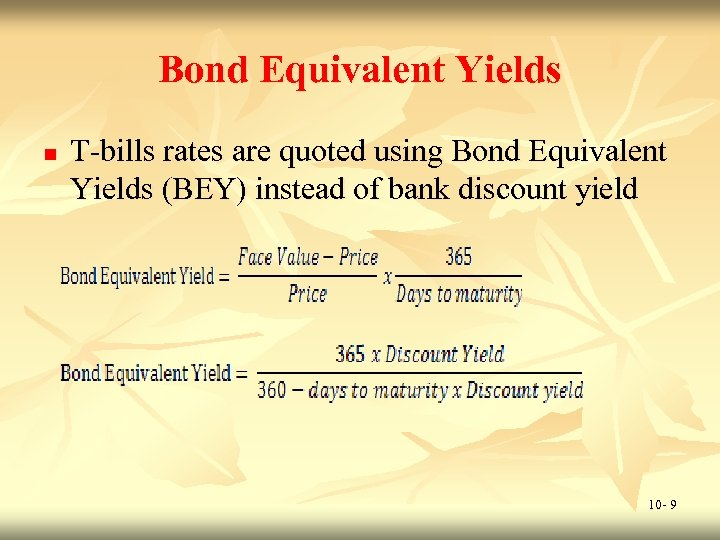Bond Equivalent Yields n T-bills rates are quoted using Bond Equivalent Yields (BEY) instead of bank discount yield 10 - 9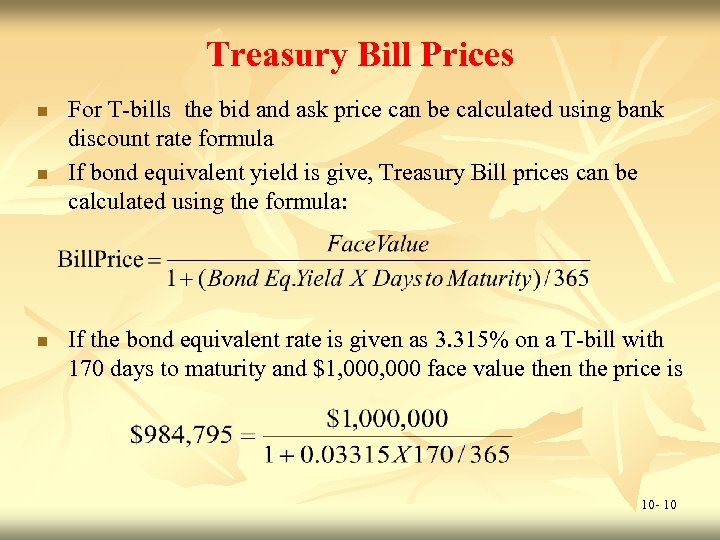Treasury Bill Prices n n n For T-bills the bid and ask price can be calculated using bank discount rate formula If bond equivalent yield is give, Treasury Bill prices can be calculated using the formula: If the bond equivalent rate is given as 3. 315% on a T-bill with 170 days to maturity and \$1, 000 face value then the price is 10 - 10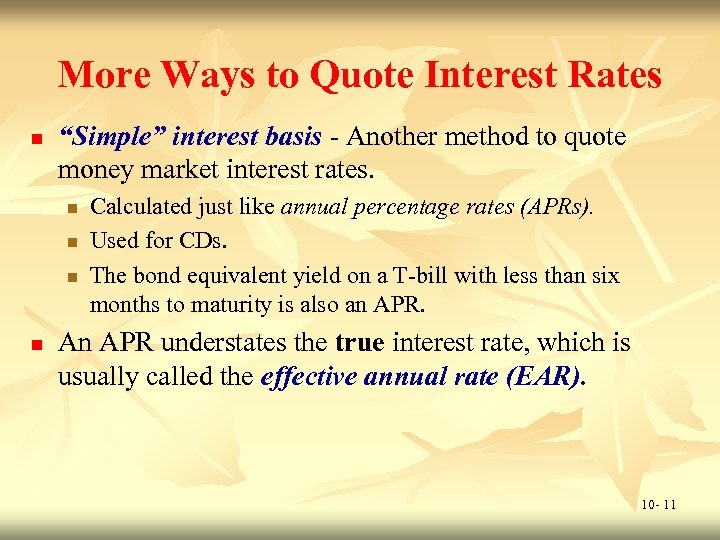More Ways to Quote Interest Rates n “Simple” interest basis - Another method to quote money market interest rates. n n Calculated just like annual percentage rates (APRs). Used for CDs. The bond equivalent yield on a T-bill with less than six months to maturity is also an APR. An APR understates the true interest rate, which is usually called the effective annual rate (EAR). 10 - 11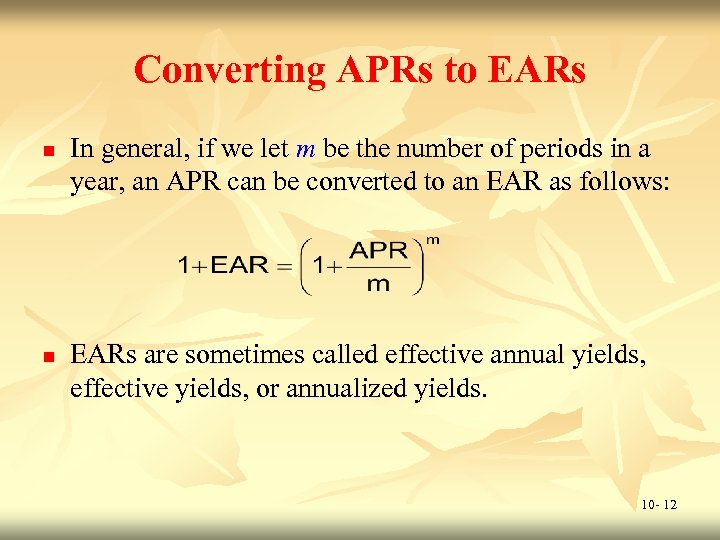Converting APRs to EARs n n In general, if we let m be the number of periods in a year, an APR can be converted to an EAR as follows: EARs are sometimes called effective annual yields, effective yields, or annualized yields. 10 - 12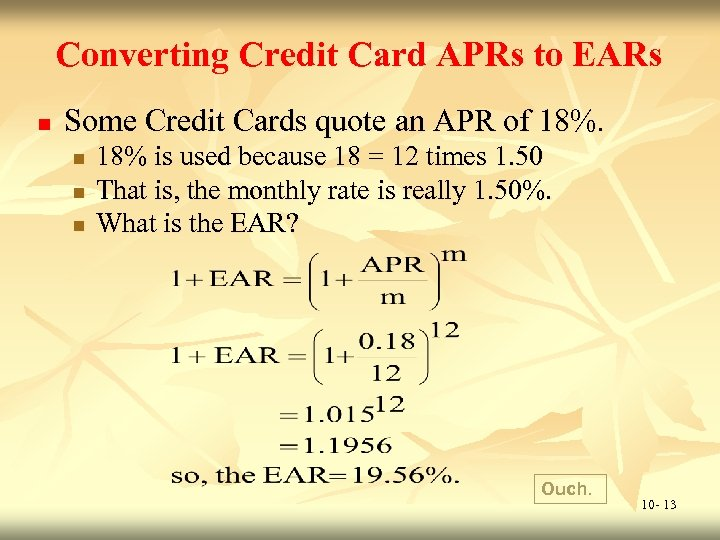Converting Credit Card APRs to EARs n Some Credit Cards quote an APR of 18%. n n n 18% is used because 18 = 12 times 1. 50 That is, the monthly rate is really 1. 50%. What is the EAR? Ouch. 10 - 13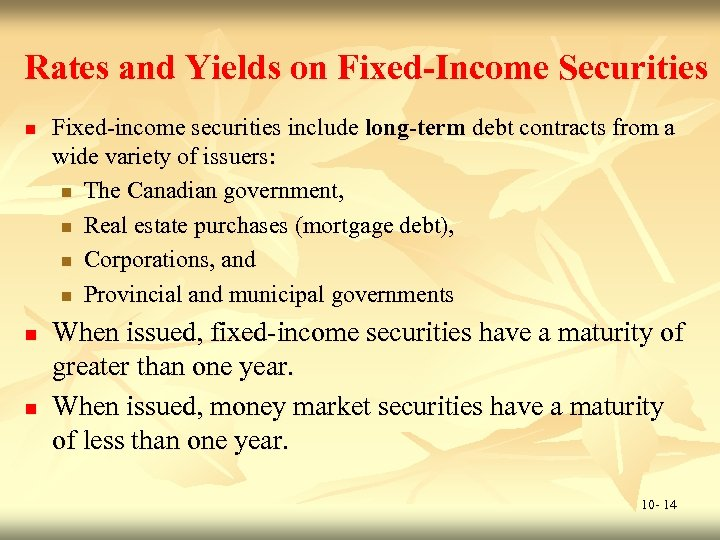Rates and Yields on Fixed-Income Securities n n n Fixed-income securities include long-term debt contracts from a wide variety of issuers: n The Canadian government, n Real estate purchases (mortgage debt), n Corporations, and n Provincial and municipal governments When issued, fixed-income securities have a maturity of greater than one year. When issued, money market securities have a maturity of less than one year. 10 - 14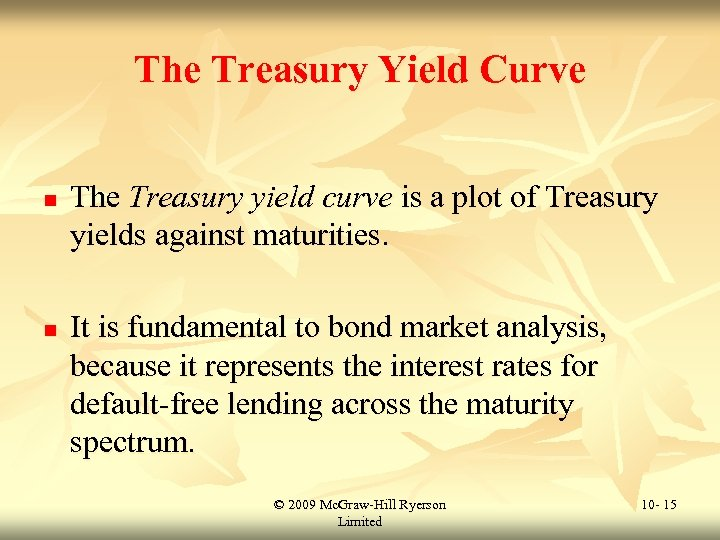The Treasury Yield Curve n n The Treasury yield curve is a plot of Treasury yields against maturities. It is fundamental to bond market analysis, because it represents the interest rates for default-free lending across the maturity spectrum. © 2009 Mc. Graw-Hill Ryerson Limited 10 - 15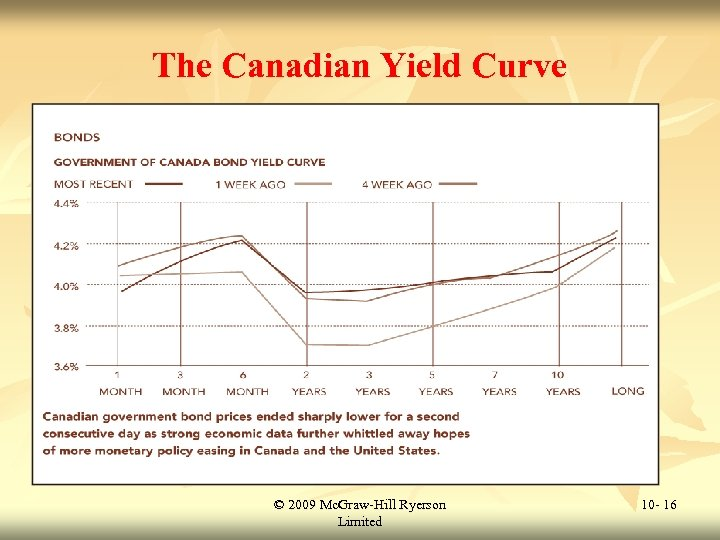The Canadian Yield Curve © 2009 Mc. Graw-Hill Ryerson Limited 10 - 16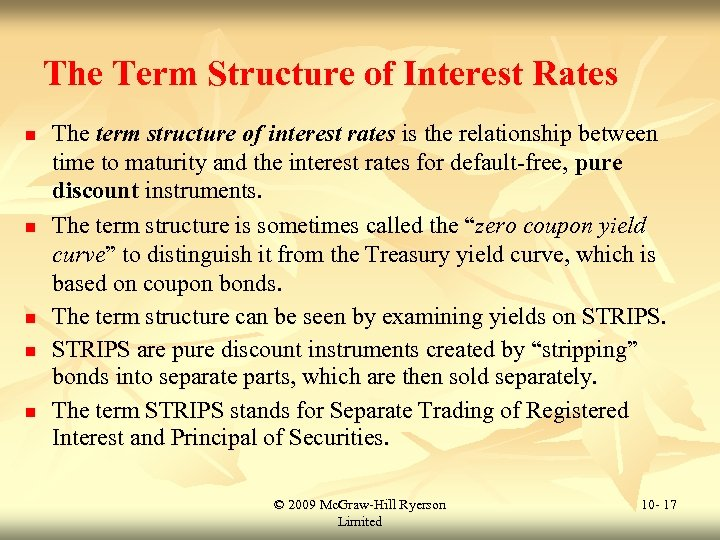The Term Structure of Interest Rates n n n The term structure of interest rates is the relationship between time to maturity and the interest rates for default-free, pure discount instruments. The term structure is sometimes called the “zero coupon yield curve” to distinguish it from the Treasury yield curve, which is based on coupon bonds. The term structure can be seen by examining yields on STRIPS are pure discount instruments created by “stripping” bonds into separate parts, which are then sold separately. The term STRIPS stands for Separate Trading of Registered Interest and Principal of Securities. © 2009 Mc. Graw-Hill Ryerson Limited 10 - 17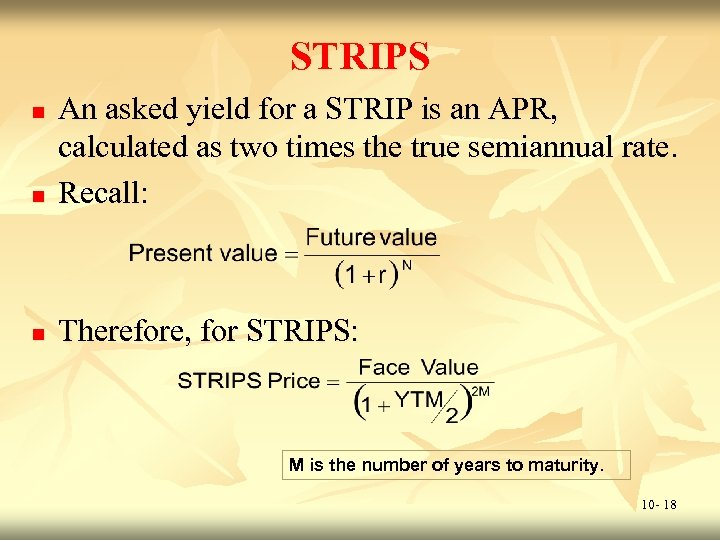STRIPS n An asked yield for a STRIP is an APR, calculated as two times the true semiannual rate. Recall: n Therefore, for STRIPS: n M is the number of years to maturity. 10 - 18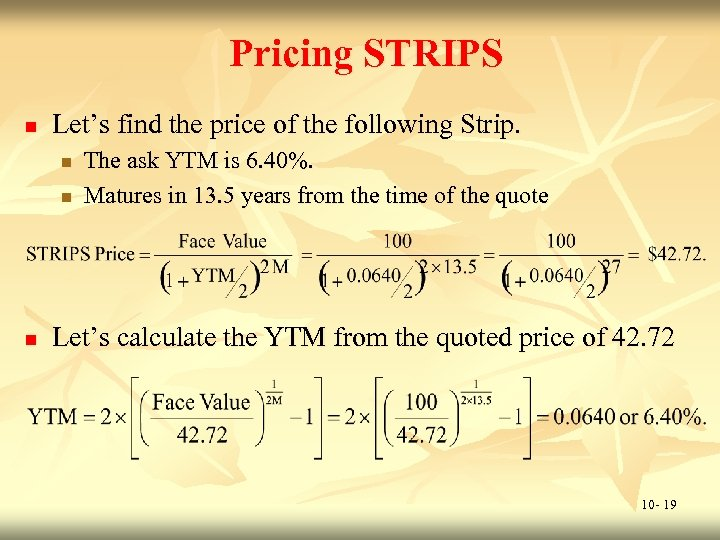Pricing STRIPS n Let’s find the price of the following Strip. n n n The ask YTM is 6. 40%. Matures in 13. 5 years from the time of the quote Let’s calculate the YTM from the quoted price of 42. 72 10 - 19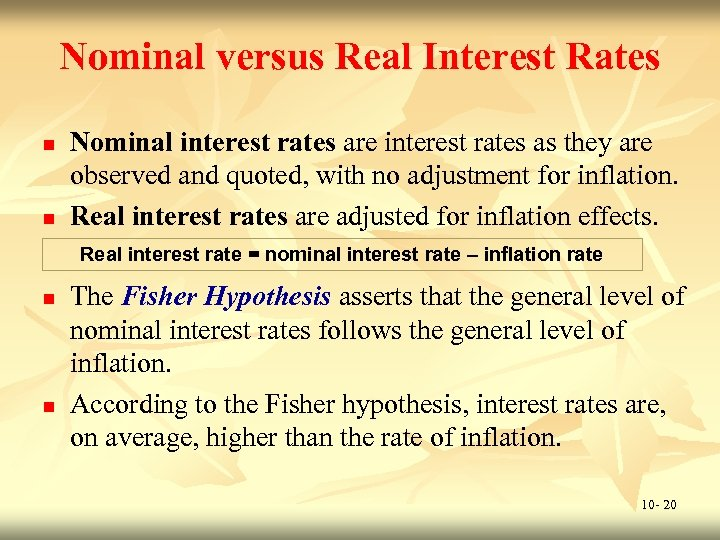Nominal versus Real Interest Rates n n Nominal interest rates are interest rates as they are observed and quoted, with no adjustment for inflation. Real interest rates are adjusted for inflation effects. Real interest rate = nominal interest rate – inflation rate n n The Fisher Hypothesis asserts that the general level of nominal interest rates follows the general level of inflation. According to the Fisher hypothesis, interest rates are, on average, higher than the rate of inflation. 10 - 20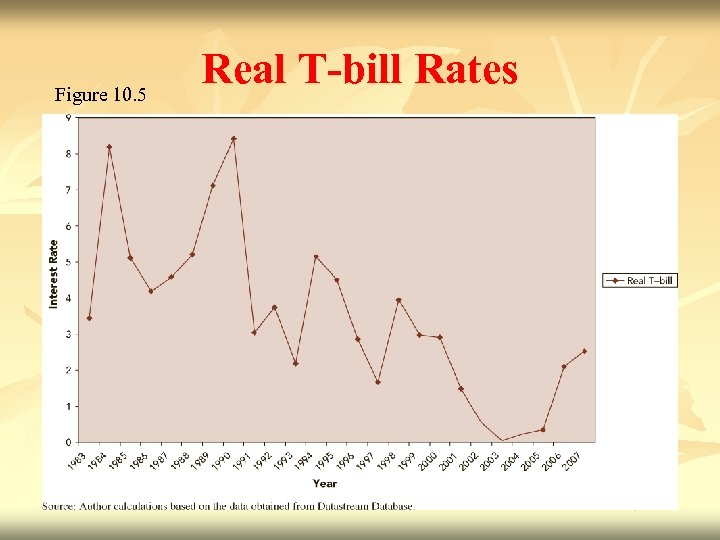Figure 10. 5 Real T-bill Rates 10 - 21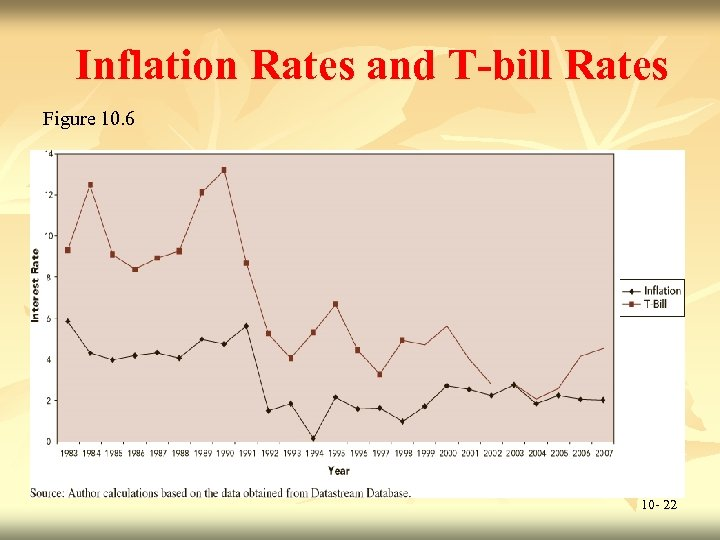Inflation Rates and T-bill Rates Figure 10. 6 10 - 22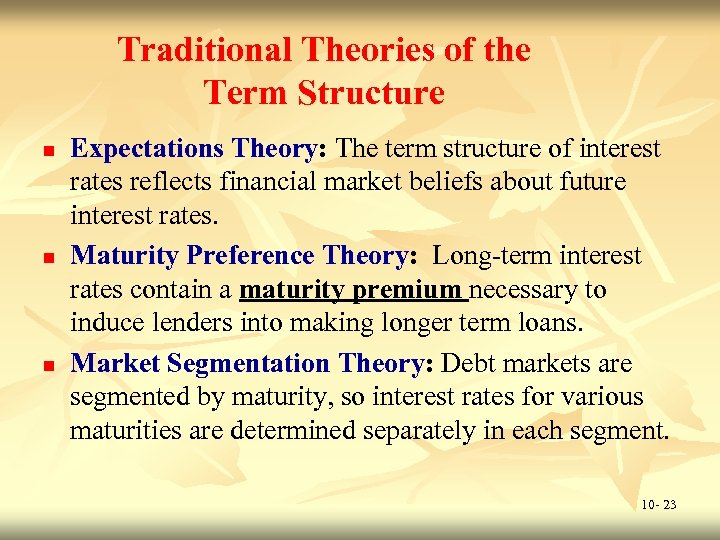Traditional Theories of the Term Structure n n n Expectations Theory: The term structure of interest rates reflects financial market beliefs about future interest rates. Maturity Preference Theory: Long-term interest rates contain a maturity premium necessary to induce lenders into making longer term loans. Market Segmentation Theory: Debt markets are segmented by maturity, so interest rates for various maturities are determined separately in each segment. 10 - 23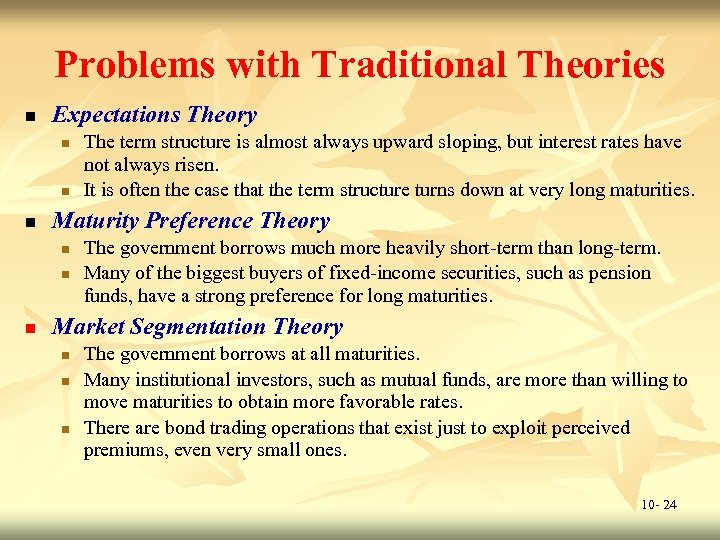Problems with Traditional Theories n Expectations Theory n n n Maturity Preference Theory n n n The term structure is almost always upward sloping, but interest rates have not always risen. It is often the case that the term structure turns down at very long maturities. The government borrows much more heavily short-term than long-term. Many of the biggest buyers of fixed-income securities, such as pension funds, have a strong preference for long maturities. Market Segmentation Theory n n n The government borrows at all maturities. Many institutional investors, such as mutual funds, are more than willing to move maturities to obtain more favorable rates. There are bond trading operations that exist just to exploit perceived premiums, even very small ones. 10 - 24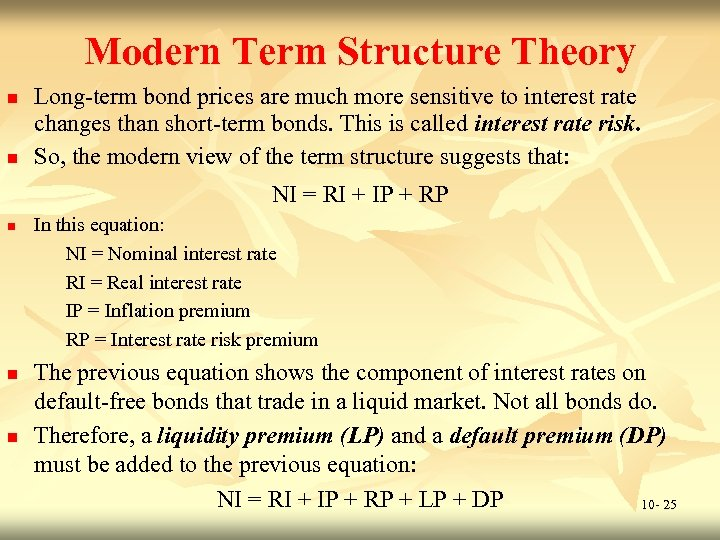Modern Term Structure Theory n n Long-term bond prices are much more sensitive to interest rate changes than short-term bonds. This is called interest rate risk. So, the modern view of the term structure suggests that: NI = RI + IP + RP n n n In this equation: NI = Nominal interest rate RI = Real interest rate IP = Inflation premium RP = Interest rate risk premium The previous equation shows the component of interest rates on default-free bonds that trade in a liquid market. Not all bonds do. Therefore, a liquidity premium (LP) and a default premium (DP) must be added to the previous equation: NI = RI + IP + RP + LP + DP 10 - 25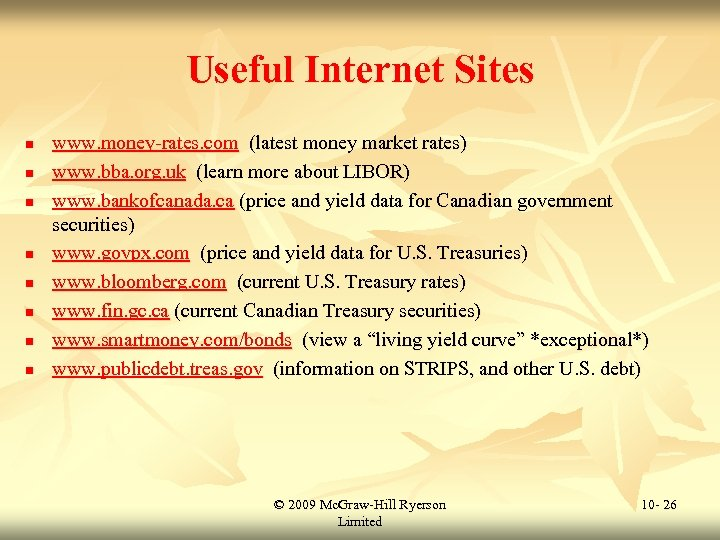Useful Internet Sites n n n n www. money-rates. com (latest money market rates) www. bba. org. uk (learn more about LIBOR) www. bankofcanada. ca (price and yield data for Canadian government securities) www. govpx. com (price and yield data for U. S. Treasuries) www. bloomberg. com (current U. S. Treasury rates) www. fin. gc. ca (current Canadian Treasury securities) www. smartmoney. com/bonds (view a “living yield curve” *exceptional*) www. publicdebt. treas. gov (information on STRIPS, and other U. S. debt) © 2009 Mc. Graw-Hill Ryerson Limited 10 - 26